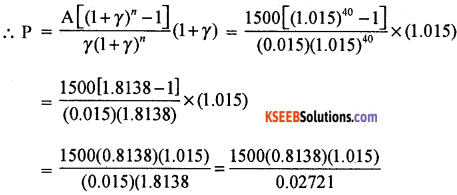1st PUC Basic Maths Question Bank Chapter 9 Annuities

Students can Download Basic Maths Chapter 9 Annuities Questions and Answers, Notes Pdf, 1st PUC Basic Maths Question Bank with Answers helps you to revise the complete Karnataka State Board Syllabus and score more marks in your examinations.

Karnataka 1st PUC Basic Maths Question Bank Chapter 9 Annuities

Question 1.
A company needs Rs. 10,00,000 at the end of 5 years from now. It would like to set aside an equal amount each year out of its profits. If the present marker rate of interest is 16%, how much should be annual amount to be invested?
It is under future value of annuity immediate
Given future value of annuity (F) = Rs. 10,00,000
Number of years = 5, r = 16%
∴ We have to find the value of annuity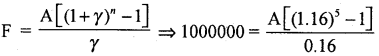⇒ 10,00,000 × 0.16 = A[(1.16)5 – 1]
1,60,000 = A[2.0990 – 1]
1.099 A = 1,60,000
∴ A = $$\frac{160000}{1.099}$$ = 1,45,586.90
Calculate (1.16)5
(5 log to 6 = AL (5 × 0.00644)
= AL)0.322) = 2.990
The company set aside Rs. 1,45,586.90 each year to get Rs. 10,00,000 at the end of 5 years.Question 2.
You have taken a loan of Rs. 10,000 from a finance company. Under the agreement you have to repay the loan in 5 equal instalments commencing from the end of year. If the finance company charge you interest of 18% p.a. What is the value of instalment? Also show that at the end of 5 years your loan account will be closed.
It is under present value of annuity immediate Rs. 10,000 be the instalment which include principal and interest. Since the instalments are = equal.
(a) To find the instalment ‘A’, we have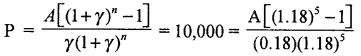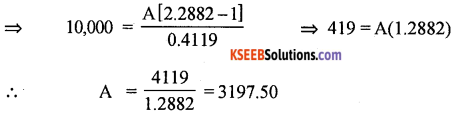(b) Loan repayment statement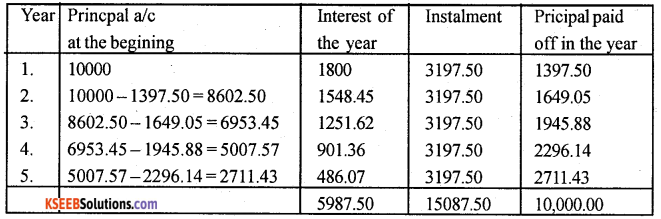Question 3.
Bank of India offers 12% p.a. interest on 12 months R.D a/c’s. If v deposit Rs. 1000 p.a. for 12 months how much can I get at the end of 12 months, assuming that each deposit is to be made of the begining of the month?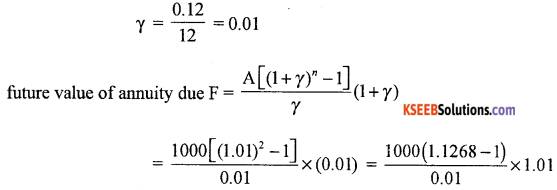r = $$\frac { 0.18 }{ 12 }$$ = 0.15 n = 40 months A = Rs.1500